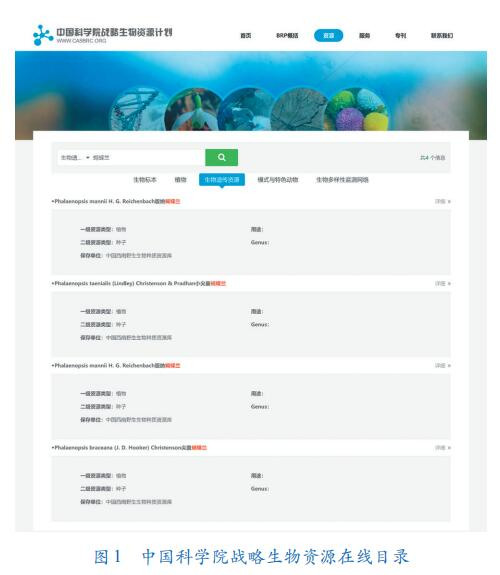Python有个处理大数据的库，结合xlrd库，在做一些大数据的处理统计工作的时候很好用，譬如做性能测试，你的结果数据如何统计，python有个库pandas，这个就很擅长做这个工作，这里就讲2个pandas的神操作。 pandas中groupby、Grouper和agg函数的使用。这2个函数作用类似，都是对数据集中的一类属性进行聚合操作，比如统计一个用户在每个月内的全部花销，统计某个属性的最大、最小、累和、平均等数值。importpandasaspd

importcollections

df["date"]=pd.to_datetime(df["date"])

df1=df.set_index("date").resample("M")['extprice'].sum()df2=df.groupby(["name",pd.Grouper(key="date",freq="M")])["extprice"]Agg

agg函数，它提供基于列的聚合操作。而groupby可以看做是基于行，或者说index的聚合操作。

df3=df[["extprice","quantity","unitprice"]].agg(["sum","mean"])df4=df.agg({"extprice":["sum","mean"],"quantity":["sum","mean"],"unitprice":["mean"]})#统计sku中，购买次数最多的产品编号

get_max=lambdax:x.value_counts(dropna=False).index

get_max.__name__="mostfrequent"

df5=df.agg({"extprice":["sum","mean"],

"quantity":["sum","mean"],

"unitprice":["mean"],

"sku":[get_max]

})

print(df5)agg_dict={

"extprice":["sum","mean"],

"quantity":["sum","mean"],

"unitprice":["mean"],

"sku":[get_max]

}

#按照列名的长度排序。OrderedDict的顺序是跟插入顺序一致的

df6=df.agg(collections.OrderedDict(sorted(agg_dict.items(),key=lambdax:len(x))))

print(df6)#### 推荐内容

•• ##### 2020年预测 人工智能和数据发展趋势

•• ##### 大数据“联姻”搜索引擎优化 企业或将“战无不胜”

•##### 大数据人才缺口巨大 大数据分析师VS大数据工程师

•• ##### 大数据时代来了 “加减乘除”算术题要做好

•• ##### 全面提升现代化管理手段，高分数据已得到广泛应用

•• ##### 人工智能和大数据携手并进 生活大改变渐行渐近

•• ##### 贵州：“大数据+监督” “让监督插上科技翅膀”

•##### “曲线”出行—大数据透视2020年春节人口流动趋势

•##### “数据密集型科学”转变 生物资源大数据及应用提上日程

•##### 减少企业数据开发难点 大数据平台来助力

•• ##### 城市大数据=城市数据+大数据技术+城市职能

•##### 交通运输部发布 推进综合交通运输大数据发展行动纲要

•##### 大数据时代 数据挖掘+数据分析已成“绝技”

•